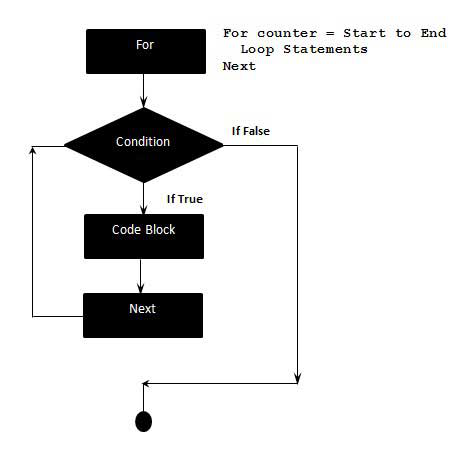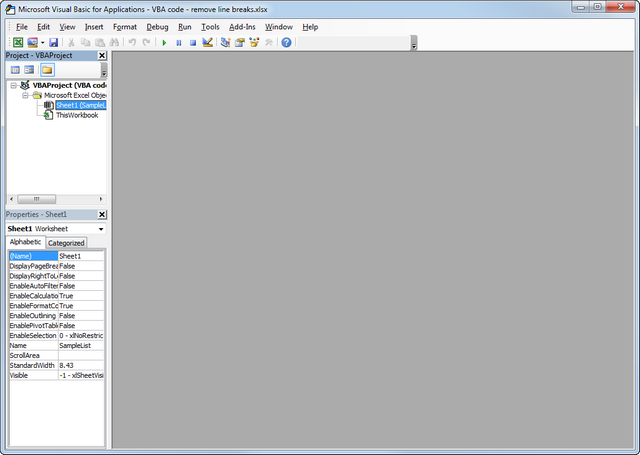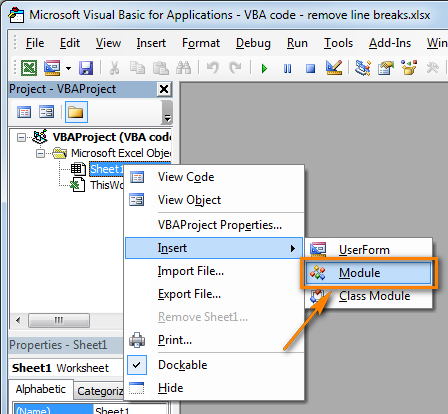# How to Use For Next Loop in Excel VBA?

• Difficulty Level : Hard
• Last Updated : 13 Jun, 2022

If you are familiar with the programming you must have an idea of what a loop is, In computer programming, a loop is a sequence of statements that are repeated until a specific condition is satisfied.

In Excel VBA the “For Next” loop is used to go through a block of code a specific number of times.

Syntax:

``` For counter = start to end [step count]

statement 1
statement 2
statement 3
.
.
statement n
Next [counter]```

Here we can use the counter or any other variable to run them as many times as we need.

Example:

When you are displaying numbers from 1 to 10 you may want to set the value of a variable to 1 and display it 10 times, increasing its value by 1 on each loop iteration. The same logic is used in VBA.

We specify how many times we have to run the loop, and then specify what code should our loop execute each time the loop runs.

A loop has 3 parts the first one is an initialization, the second is the condition under which the loop runs, and the last is increment or decrement.

### The flow of the control in for loop:

• The For step is executed first. This step allows you to initialize any loop control variables and increment the step counter variable.
• Then the second step is, the condition is evaluated. If it is true, the body of the loop is executed. If it is false, the body of the loop does not execute and the flow of control jumps to the next statement, just after the For Loop.
• After the body of the For loop executes, the flow of control jumps to the next statement. This statement allows you to update any loop control variables. .the value is updated as the value we write in the count.
• The condition is evaluated again it continues till the condition becomes false.Flow Diagram

Now let us look at an example to understand how the for loop works. Follow the below steps to work along with this article:

Step 1: Press Alt + F11 to open the VBA(visual basic editor where we can write the code).Step 2: Right-click on the workbook name and then insert a module.Note: The interface of Excel may change depending upon your version.

STEP 3: Once a module is inserted we can write our code. Let's say we are writing code of print sum  of first 10 numbers so our code will be as follows:

```Sub Sumnumbers()
Dim Total as Integer
Dim Count as Integer
Total = 0                   //initialised total as 0
For count = 1 to 10 //
Total = Total + count //
Next count //increments counter N = N + 1
MsgBox Total //prints total sum
End Sub   // code ended```

So, what's happening in the above code:

• Here in the first line, we write the subject of code this is mostly a point where our code starts sub is also important as it breaks large pieces of code in small parts.
• As we use to declare in our normal programming we declare two integers the first one is total where we will store the sum and the other is count to count n variables in a loop
• The "for next" loop starts here from count =1 and will run till the count is equal to 10.
• Then we will evaluate the total count by adding the count to the total:
`Total = Total + count`
• Then we increment the counter by 1 and repeat the above operations till the count is 10.
• At the end of the loop, we will show the output using a message box.
• Finally, we will end the subject.

Output:

`55`

Example 2: Printing product of odd +ve integers till 10

```Sub ProductNumber()
Dim Product as Integer
Dim Total as Integer
Product = 1
For Count 1 to 10 Step 2   // when we use step 2 it tells compiler to increment
// count  by +2 such as 1,3,5,7,..
Product = Product * count
Next Count
Msgbox Product
End Sub```

Output:

`12150`
My Personal Notes arrow_drop_up
Recommended Articles
Page :